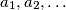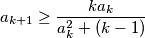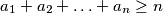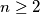IMO Shortlist 2015 problem A1

Kvaliteta:
Avg: 0,0
Težina:
Avg: 6,0

Suppose that a sequence$a_1,a_2,\ldots$ of positive real numbers satisfiesfor every positive integer$k$. Prove that$a_1+a_2+\ldots+a_n\geq n$ for every$n\geq2$.

(Serbia)

Izvor: https://www.imo-official.org/problems/IMO2015SL.pdf